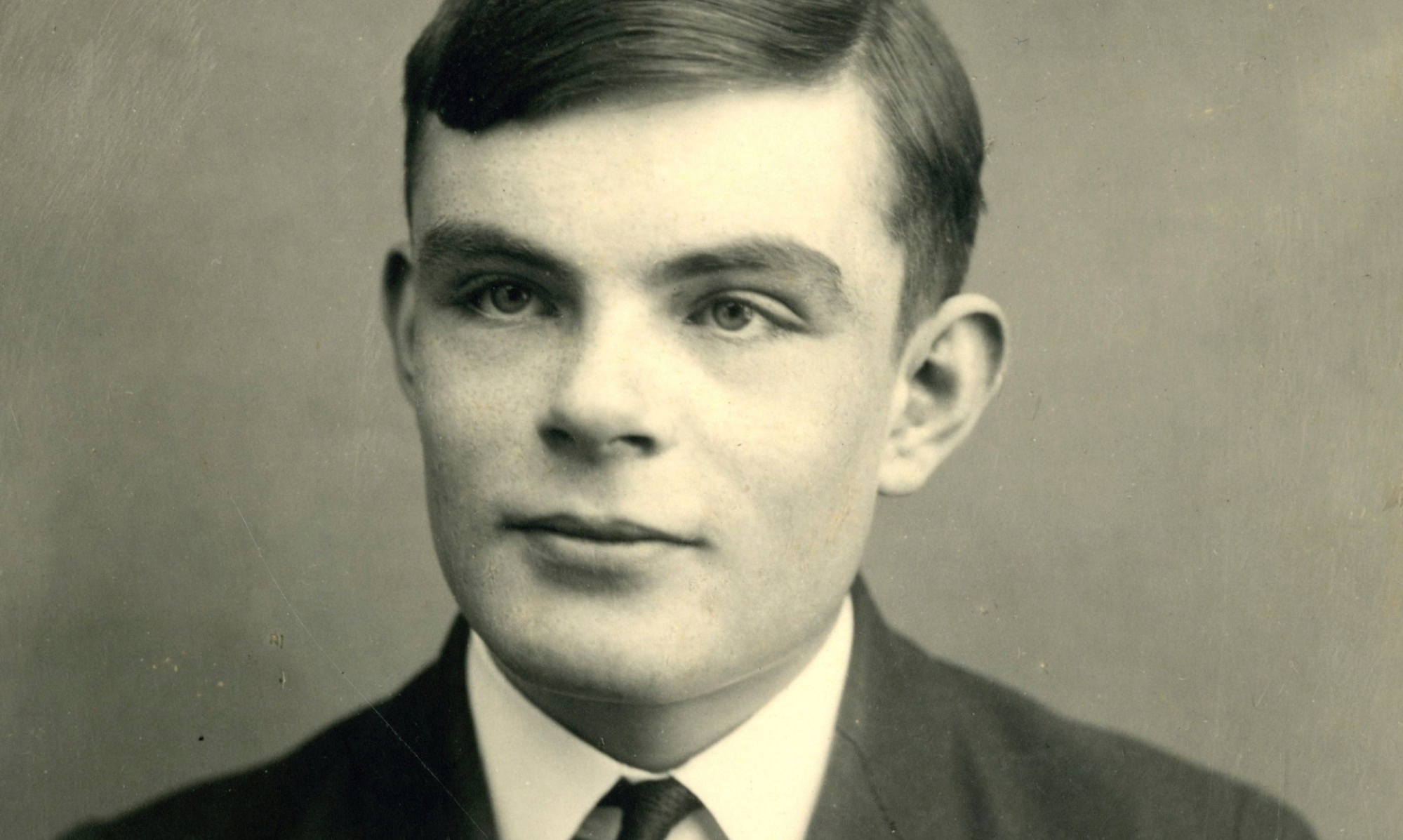### CSCI 5444 - Trivedi - Introduction to Theory of Computation

The objective of this course is provide an introduction to the theory of computation covering the following three branches of theoretical computer science:
1. Automata Theory
• Formalization of the notion of problems via formal languages
• Formalization of the notion of computation using "abstract computing devices" called automata
• Understanding a hierarchy of classes of problems or formal languages (regular, context-free, context-sensitive, decidable, and undecidable)
• Understanding a hierarchy of classes of automata (finite automata, pushdown automata, and Turing machines)
• Understanding applications to pattern matching, parsing, and programming languages
2. Computability Theory
• Understanding Church-Turing thesis (Turing machines as a notion of "general-purpose computers")
• Understanding the concept of reduction , i.e., solving a problem using a solution (abstract device) for a different problem
• Understanding the concept of undecidability , i.e., when a problem can not be solved using computers
3. Complexity Theory
• Complexity classes : how to classify decidable problems based on their time and space requirements
• Complexity classes P and NP
• When a problem is called intractable (NP-completeness)
• Using reductions to prove problems intractable